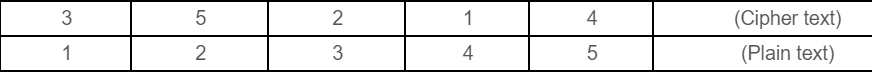91. A slotted ALOHA network transmits 200 bits frames using a shared channel with 200 kbps bandwidth. If the system (all stations put together) produces 1000 frames per second, then the throughput of the system is ................. a. 0.268 b. 0.468 c. 0.368 d. 0.568

 92. An analog signal has a bit rate of 8000 bps and a baud rate of 1000.Then analog signal has .............. signal elements and carry ............ data elements in each signal. a. 256, 8 bits b. 128, 4 bits c. 256, 4 bits d. 128, 8 bits

 93. The plain text message BAHI encrypted with RSA algorithm using e=3, d=7 and n=33 and the characters of the message are encoded using the values 00 to 25 for letters A to Z. Suppose character by character encryption was implemented. Then, the Cipher Text message is ............ a. ABHI b. HAQC c. IHBA d. BHQC

 94. If link transmits 4000 frames per second and each slot has 8 bits, the transmission rate of circuit of this TDM is ............... a. 64 Kbps b. 32 Mbps c. 32 Kbps d. 64 Mbps

 95. Given the following statements:(a) Frequency Division Multiplexing is a technique that can be applied when the bandwidth of a link is greater than combined bandwidth of signals to be transmitted.(b) Wavelength Division Multiplexing (WDM) is an analog multiplexing Technique to combine optical signals.(c) WDM is a Digital Multiplexing Technique.(d) TDM is a Digital Multiplexing Technique.Which of the following is correct? a. (a), (b), (c) and (d) are true b. (a), (b), (c) and (d) are false c. (a), (b) and (d) are false; (c) is true d. (a), (b) and (d) are true; (c) is false
 Answer: (d).(a), (b) and (d) are true; (c) is false

 96. A pure ALOHA Network transmit 200 bit frames using a shared channel with 200 Kbps bandwidth. If the system (all stations put together) produces 500 frames per second, then the throughput of the system is .............. a. 0.384 b. 0.184 c. 0.286 d. 0.586

 97. Match the following:(a) Line coding                         (i) A technique to change analog signal to digital data.(b) Block coding                       (ii) Provides synchronization without increasingnumber of bits(c) Scrambling                          (iii) Process of converting digital data to digital signal.(d) Pulse code modulation    (iv) Provides redundancy to ensure synchronizationand inherits error detection.Codes:      (a)   (b)   (c)   (d) a. (iv)  (iii)   (ii)   (i) b. (iii)  (iv)   (ii)   (i) c. (i)    (iii)   (ii)   (iv) d. (ii)   (i)    (iv)   (iii)
 99. Encrypt the plain text Message “EXTRANET” using Transposition cipher technique with the following key. Using ‘Z’ as bogus character.a. TAXERTZENZ b. EXTRANETZZ c. EZXZTRZANZET d. EXTZRANZETZ